矩阵分解之SVD奇异值分解

SVD的原理

矩阵相关知识

• 正交矩阵：若一个方阵其行与列皆为正交的单位向量，则该矩阵为正交矩阵，且该矩阵的转置和其逆相等。两个向量正交的意思是两个向量的内积为 0。
• 正定矩阵：如果对于所有的非零实系数向量 z，都有 $z^T \mathbf Az>0$，则称矩阵$\mathbf A$是正定的。正定矩阵的行列式必然大于0，所有特征值也必然>0。相对应的，半正定矩阵的行列式必然 ≥ 0。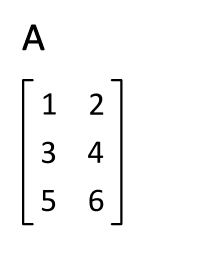SVD奇异值分解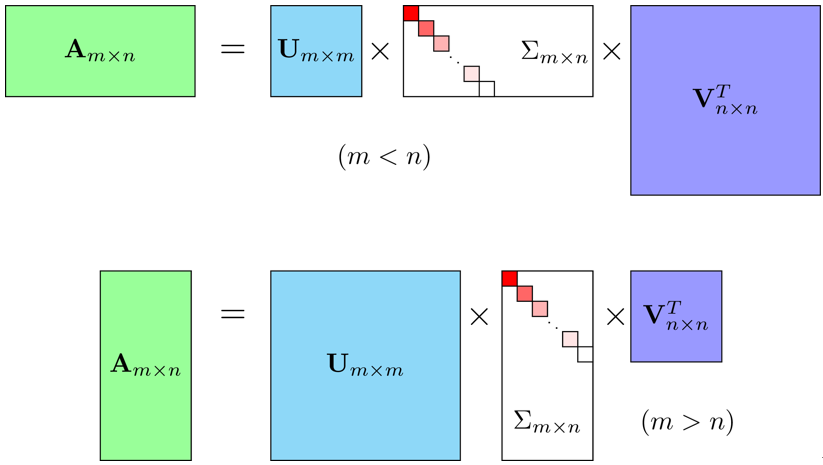$$M_{m×n}=U_{m×m} \Sigma_{m×n} V^T_{n×n}$$

$$\mathbf M = \sum_{i = 1}^{\min(m, n)}\sigma_i\vec u_i\vec v_i^{\mathsf T}$$

SVD 的计算方法

SVD 与特征值

\begin{aligned} \mathbf M\mathbf M^{\mathsf H} &{}= \mathbf U\mathbf\Sigma\mathbf V^{\mathsf H}\mathbf V\mathbf\Sigma^{\mathsf H}\mathbf U^{\mathsf H} = \mathbf U(\mathbf\Sigma\mathbf\Sigma^{\mathsf H})\mathbf U^{\mathsf H}\\ \mathbf M^{\mathsf H}\mathbf M &{}= \mathbf V\mathbf\Sigma^{\mathsf H}\mathbf U^{\mathsf H}\mathbf U\mathbf\Sigma\mathbf V^{\mathsf H} = \mathbf V(\mathbf\Sigma^{\mathsf H}\mathbf\Sigma)\mathbf V^{\mathsf H}\\ \end{aligned}

• 计算 $\mathbf M\mathbf M^{\mathsf H}$ 和 $\mathbf M^{\mathsf H}\mathbf M$；
• 分别计算$\mathbf M\mathbf M^{\mathsf H}$和$\mathbf M^{\mathsf H}\mathbf M$的特征向量及其特征值；
• $\mathbf M\mathbf M^{\mathsf H}$的特征向量组成$\mathbf U$；而$\mathbf M^{\mathsf H}\mathbf M$的特征向量组成 $\mathbf V$；
• 对$\mathbf M\mathbf M^{\mathsf H}$和$\mathbf M^{\mathsf H}\mathbf M$的非零特征值求平方根，对应上述特征向量的位置，填入$\mathbf\Sigma$的对角元。

$$\mathbf W = \mathbf M\mathbf M^{\mathsf H} = \begin{bmatrix}2 & 4 \\ 1 & 3 \\ 0 & 0 \\ 0 & 0\end{bmatrix}\begin{bmatrix}2 & 1 & 0 & 0 \\ 4 & 3 & 0 & 0\end{bmatrix} = \begin{bmatrix}20 & 14 & 0 & 0 \\ 14 & 10 & 0 & 0 \\ 0 & 0 & 0 & 0 \\ 0 & 0 & 0 & 0\end{bmatrix}$$

$$\begin{bmatrix} 20 – \lambda & 14 & 0 & 0 \\ 14 & 10 – \lambda & 0 & 0 \\ 0 & 0 & -\lambda & 0 \\ 0 & 0 & 0 & -\lambda \end{bmatrix}\vec x = \vec 0$$

$$\begin{vmatrix} 20 – \lambda & 14 & 0 & 0 \\ 14 & 10 – \lambda & 0 & 0 \\ 0 & 0 & -\lambda & 0 \\ 0 & 0 & 0 & -\lambda \end{vmatrix} = \begin{vmatrix} 20 – \lambda & 14 \\ 14 & 10 – \lambda \\ \end{vmatrix}\begin{vmatrix} -\lambda & 0 \\ 0 & -\lambda \\ \end{vmatrix} = 0$$

$$\mathbf U = \begin{bmatrix}-0.82 & -0.58 & 0 & 0 \\ -0.58 & 0.82 & 0 & 0 \\ 0 & 0 & 1 & 0 \\ 0 & 0 & 0 & 1\end{bmatrix}$$

$$\mathbf V = \begin{bmatrix}-0.40 & -0.91 \\ -0.91 & 0.40\end{bmatrix}$$

$$\mathbf\Sigma = \begin{bmatrix}5.46 & 0 \\ 0 & 0.37 \\ 0 & 0 \\ 0 & 0\end{bmatrix}$$

$$\begin{bmatrix}2 & 4 \\ 1 & 3 \\ 0 & 0 \\ 0 & 0\end{bmatrix} \approx \begin{bmatrix}-0.82 & -0.58 & 0 & 0 \\ -0.58 & 0.82 & 0 & 0 \\ 0 & 0 & 1 & 0 \\ 0 & 0 & 0 & 1\end{bmatrix}\begin{bmatrix}5.46 & 0 \\ 0 & 0.37 \\ 0 & 0 \\ 0 & 0\end{bmatrix}\begin{bmatrix}-0.40 & -0.91 \\ -0.91 & 0.40\end{bmatrix}$$

SVD的应用实战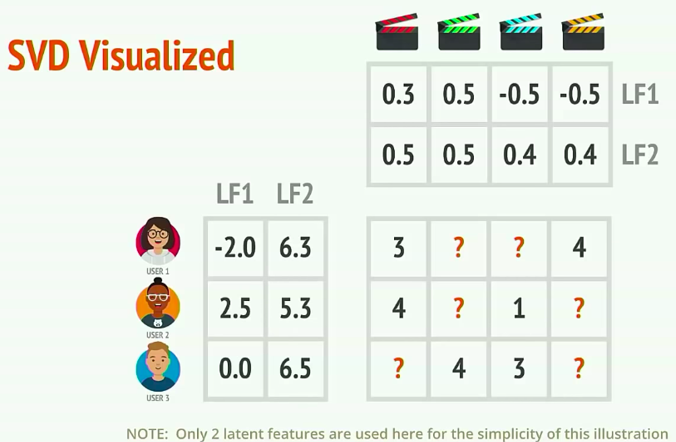1、安装Python推荐系统库：Surprise（Simple Python Recommendation System Engine）

pip install scikit-surprise

2、准备训练数据

Surprise自带数据集就支持movieslen，运行如下代码：

from surprise import Dataset
# 加载movielens数据

Dataset ml-100k could not be found. Do you want to download it? [Y/n]

from surprise import SVD
from surprise import Dataset
from surprise.model_selection import cross_validate, train_test_split
from surprise import accuracy

# 拆分训练集与测试集，75%的样本作为训练集，25%的样本作为测试集
# 这里的train_set的类型是surprise.dataset.Trainset类型，我们可以查看数据的基本信息
# train_set.n_users
# Out: 943
# train_set.n_items
# Out: 1637
# 这说明我们要用于训练的样本共有943个用户，1637个商品。

train_set, test_set = train_test_split(data, test_size=.25)

# 训练模型，指定有35个隐含特征，使用训练集进行训练
# 35隐含特征是指，原本943*1637的矩阵会被拆分成943*35和35*1637的两个矩阵乘积。
# n_factors值可以任意指定只要不超过943即可，但是设置不同的值将会拟合出不同的模型，需要选择使结果较优的值。
# n_factors我一般选择ceiling(m*n,1/4)用来测试

model = SVD(n_factors=35)

# 5折验证，输出结果
# 输出的内容为：
# Evaluating RMSE, MAE of algorithm SVD on 5 split(s).
#                   Fold 1  Fold 2  Fold 3  Fold 4  Fold 5  Mean    Std
# RMSE (testset)    0.9310  0.9320  0.9364  0.9329  0.9402  0.9345  0.0034
# MAE (testset)     0.7327  0.7357  0.7387  0.7357  0.7375  0.7361  0.0020
# Fit time          4.93    4.36    4.27    4.14    4.30    4.40    0.27
# Test time         0.24    0.31    0.30    0.20    0.19    0.25    0.05
cross_validate(model, data, measures=['RMSE', 'MAE'], cv=5, verbose=True)

# 使用训练集进行训练
model.fit(train_set)

# 模型训练完成后也可以查看拆分出来的两个矩阵
# model.pu.shape
# Out: (943, 35)
# model.qi.shape
# Out: (1637, 35)

# 使用测试集进行测试
# 输出的内容为：RMSE: 0.9413
predictions = model.test(test_set)
accuracy.rmse(predictions)

uid = str(196)  # raw user id (as in the ratings file). They are **strings**!
iid = str(302)  # raw item id (as in the ratings file). They are **strings**!

# 获取指定用户和电影的评级结果.
# 输出内容：
# user: 196        item: 302        r_ui = 4.00   est = 4.05   {'was_impossible': False}
pred = model.predict(uid, iid, r_ui=4, verbose=True)

SVD的缺点

SVD分解是早期推荐系统研究常用的矩阵分解方法，不过该方法具有以下缺点，因此很难在实际系统中应用。

• 该方法首要需要用一个简单的方法补全稀松评分矩阵。一般来说，推荐系统中的评分矩阵是非常稀疏的，一般都有95%以上的元素是缺失的。而一旦补全，评分矩阵就会变成一个稠密矩阵，从而使评分矩阵的存储需要非常大的空间，这种空间的需求在实际系统中是不可能接受的。
• 该方法依赖的SVD分解方法的计算复杂度较高，特别是在稠密的大规模矩阵上更是非常慢。一般来说，这里的SVD分解用于1000维以上的矩阵就已经非常慢了，而实际系统动辄上千万的用户和几百万的物品，所以这一方法无法使用。如果仔细研究这方面的论文可以发现，实验都是在几百个用户、几百个商品的数据集上进行的。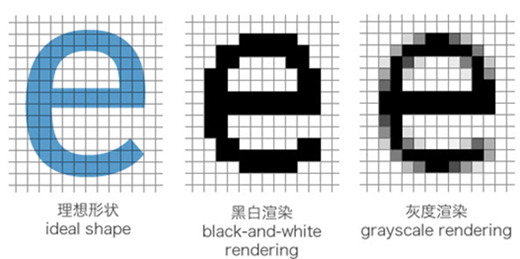网站与APP开发中的字体设置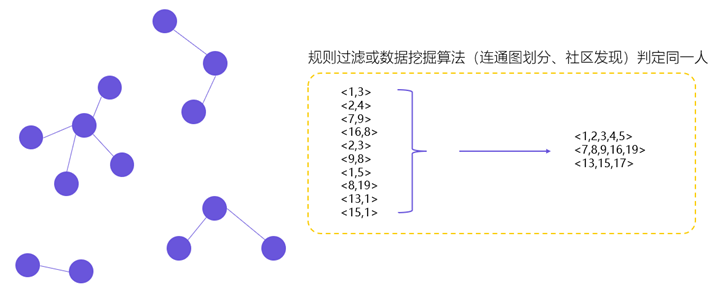用户体系搭建之ID-Mapping